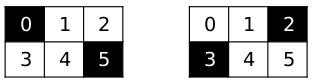# #537. 【IOI2019】视觉程序

• $\texttt{NOT}$：有唯一一个输入。若输入为 $0$ 时，其输出为 $1$，否则为 $0$。
• $\texttt{AND}$：有一个或多个输入。其输出为 $1$ 当且仅当输入全部为 $1$。
• $\texttt{OR}$：有一个或多个输入。其输出为 $1$ 当且仅当输入中至少有一个 $1$。
• $\texttt{XOR}$：有一个或多个输入。其输出为 $1$ 当且仅当输入中 $1$ 的个数是奇数。

### 实现方法

void construct_network(int H, int W, int K)
• $H,W$：机器人摄像头所拍到的图像的尺寸。
• $K$：一个正整数。
• 这个函数需要生成一个机器人的程序。对于机器人摄像头所拍到的每幅图像，该程序应判定图像中两个黑色像素之间的距离是否正好为 $K$。

int add_not(int N)
int add_xor(int[] Ns)

• $N$（对于 $\texttt{NOT}$ 而言）：要追加的 $\texttt{NOT}$ 指令的输入存储单元编号。
• $Ns$（对于 $\texttt{AND,OR,XOR}$ 而言）：要追加的 $\texttt{AND,OR}$ 或 $\texttt{XOR}$ 指令的输入存储单元的编号的数组。
• 每次函数调用都会返回追加指令的输出存储单位的编号。对这些函数的连续调用将会返回从 $H \times W$ 开始的连续整数。

• $\texttt{Instruction with no inputs}$：一个空数组被作为 $\texttt{AND,OR}$ 或 $\texttt{XOR}$ 的输入。
• $\texttt{Invalid index}$：给 $\texttt{AND,OR,XOR}$ 或 $\texttt{NOT}$ 提供了不正确（可能是负数）的存储单元编号作为输入。
• $\texttt{Too many instructions}$：你的函数尝试添加多于 $10^4$ 条的指令。
• $\texttt{Too mant inputs}$：程序中的指令一共读取了多于 $10^6$ 个值。

### 评测程序示例

• 第 $1$ 行：$H\ W\ K$
• 第 $2 \sim i$ 行 ：$r1_i\ c1_i\ r2_i\ c2_i$
• 最后一行：$-1$
• 除第一行和最后一行外，每一行都表示了一幅含有两个黑色像素的图像。 记第 $2+i$ 行上的图像为图像 $i$。该图像中，一个黑色像素位于第 $r1_i$ 行及第 $c1_i$ 列上，令一个黑色像素位于第 $r2_i$ 行及第 $c2_i$ 列上。

### 样例一$\texttt{add_and\{0,5\}}$，将加入一条指令，当且仅当图像符合情况一时其输出为 $1$ 。输出结果将保存在存储单元 $6$ 里。 $\texttt{add_and\{2,3\}}$，将加入一条指令，当且仅当图像符合情况二时其输出为 $1$。输出结果将保存在存储单元 $7$ 里。 $\texttt{add_or\{6,7\}}$，将加入一条指令，当且仅当上述两种情况之一成立时其输出为 $1$。

### 数据范围

$1$$max(H,W) \le 310 2$$max(H,W) \le 10$11
$3$$max(H,W) \le 3011 4$$max(H,W) \le 100$15
$5$$min(H,W) = 112 6保证像素(0,0)是黑色的8 7$$K=1$14
$8$无附加约定19